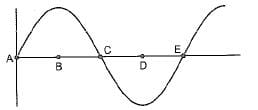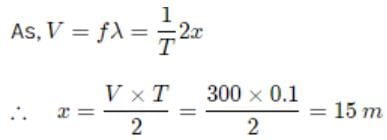# MCQ : Sound - 2

## 20 Questions MCQ Test Science & Technology for UPSC CSE | MCQ : Sound - 2

Description
Attempt MCQ : Sound - 2 | 20 questions in 20 minutes | Mock test for Class 9 preparation | Free important questions MCQ to study Science & Technology for UPSC CSE for Class 9 Exam | Download free PDF with solutions
QUESTION: 1

### Speed of light or electromagnetic wave is higher than that of the speed of sound in air by

Solution:

Speed of light = 3* 108 m/s
Speed of sound = 3* 10
Subtract  exponents to get the order.
Therefore, 106  is the correct ✔ answer.

QUESTION: 2

Solution:
QUESTION: 3

### Sonic booms are caused by the combination of

Solution:

The boom is the plane's sound waves combined together propagated at once. Air reacts like fluid to supersonic objects. As those objects travel through the air, molecules are pushed aside with great force and this forms a shock wave.

QUESTION: 4

Sound takes 2 seconds to travel 3 kilometres in water. What is the speed of sound in water?

Solution:

Here, distance = 3 km = 3000 m
Time  = 2 sec
Therefore, speed= distance / time
=  3000 /2
=  1500 m /s

QUESTION: 5

Infrasonic fi Audible fa and Ultrasonic fu sounds are related a

Solution:

Sound is only audible to the average humar ear if the frequencies lie between 20 Hz and 20 kHz. The actual range varies from person to person. Sound waves with frequencies less than 20 Hz are called infrasonic or subsonic and those with frequencies above 20 kHz are called ultrasonic

QUESTION: 6

Ultrasonic waves have frequencies

Solution:
QUESTION: 7

Which of the following is not the character of mechanical waves ?

Solution:
QUESTION: 8

Ultrasound is an application of

Solution:
QUESTION: 9

In the bell jar experiment, as air is removed

Solution:

The experiment is done by placing an electric bell in the bell jar. As the air is pumped out of the sealed bell jar, the sound from the bell jar fades. This demonstrates that the sound wave cannot travel through vacuum. That is, a sound wave needs a material medium for its propagation.

QUESTION: 10

Sound travels fastest in

Solution:
QUESTION: 11

Echo is not heard in a room of 10 m x 10 in x 10 m dimension due to (speed of sound in air = 300 m/s)

Solution:

If you have persistence, you continue to do something even though it is difficult or others are against it.

( As the sensation of sound persists in our brain for about 0.1 s, to hear a distinct echo the time interval between  the original sound and the reflected one must be at least 0.1 s. If we take the speed of the sound to be 300 m/s at a given temperature, in air, sound must go to the obstacle and reach back the ear of the listener on reflection after 0.1s. )

QUESTION: 12

A key of a mechanical piano is struck gently and then struck again but much harder this time. In tine second case

Solution:
QUESTION: 13

The instruments Megaphone, Stethoscope, Hearing aids and Sound boards work based on the principle of

Solution:

Reflection of sound is used in many devices. For example ; megaphone, loudspeaker, bulb horn, stethoscope, hearing aid, sound board etc.

QUESTION: 14

In tlhe curve shown in figure half the wavelength isSolution:
QUESTION: 15

A wave is moving with a speed of 3000 cm/s with reversal after every 0.01 second. The wavelength of the wave is

Solution:

The correct option is Option B.

Given:

T = 0.1 sec

V = 3000 m/s

V = νλ = λ/T  ( as ν = 1/T )

3000 =  λ/0.1

λ = 30 m

QUESTION: 16

Earthquake produces which kind of sound before the main shock begins?

Solution:
QUESTION: 17

Sonic booms are caused due to the variation of

Solution:
QUESTION: 18

Sound travels

Solution:

Sound  travels faster in soilds than in liquids, and faster in liquids than in gases. This is because the density of solids is higher than that of liquids which means that the particles are closer together.

QUESTION: 19

The minimum size of a room required to hear an echo of sound with a speed of 300 m/s is

Solution:

To hear a distinct echo the time interval between the original sound and the reflected one must be at least 0.15QUESTION: 20

A thunder clap is heard 5.5 seconds after lightning flash. The distance of the flash is (Velocity of sound in air = 330 m/s)

Solution:

The speed is 330 m / s and the time taken is 5.5 second. Therefore, the distance would be speed * time ⇒ 5.5 * 330 = 1815mUse Code STAYHOME200 and get INR 200 additional OFF Use Coupon Code